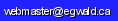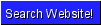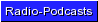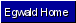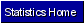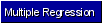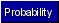Egwald Statistics: Probability and Stochastic Processes by Elmer G. Wiens Egwald's popular web pages are provided without cost to users. Please show your support by joining Egwald Web Services as a Facebook Fan:Follow Elmer Wiens on Twitter:Hypotheses Testing B. Testing the Variance The random variable S^2 defined by S^2 = [(X1 - Y)^2 + ... + (Xn - Y)^2] / (n - 1) where Y is the mean of the observations {X1, ..., Xn}, is called the sample variance. S is called the standard error of the mean. 1. Sample from a normal population The r.v. Z = (n - 1) * S^2 / ø^2 has the chi-square distribution with n - 1 degrees of freedom. To test the hypotheses H0: ø^2 = þ against H1: ø^2 != þ determine where on the chi-square density the computed value of Z falls. With a sample of 16 observations, we can reject H0, at the 95% level of confidence, if the Z statistic falls in either of the two critical regions: to the right of 27.488, or to the left of 6.262.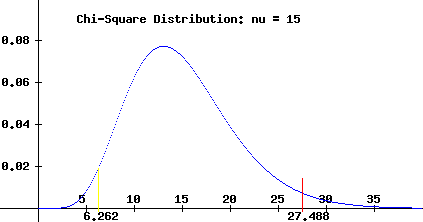2. Comparing the variances of two populations Suppose we compute sample variances S^2 and R^2 from samples of size n and m drawn from two populations. Assume that S^2 >= R^2. Then the statistic F = S^2 / R^2 has the F-distribution with (n - 1) and (m - 1) degrees of freedom. If n = 20 and m = 10, then the F19,9 distribution is:Reject the equality of the variances, at the 90% level, if the F statistic lies to the right of 2.42.Copyright © Elmer G. Wiens:   Egwald Web ServicesAll Rights Reserved.    Inquiries